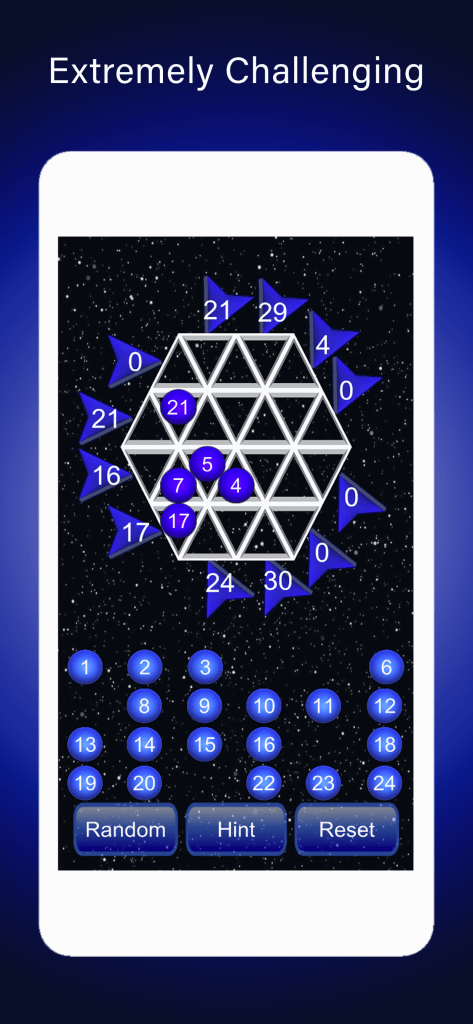## Magic Hexagon Math PuzzleThis magic hexagon game is based on Magic Squares which have fascinated mathematicians for over a thousand years.

## Magic Squares, Magic Triangles, Magic Hexagon game app

This Magic hexagon game app is a number puzzle based on magic squares and magic hexagons. Specifically there are 4 levels from fairly simple to almost impossible! Extremely challenging. Intriguingly on the hardest level there are over 50 million solutions but finding just one can be difficult. There are 59456127 ways of placing the numbers 1 – 24 in the correct order so that all the horizontal and diagonal lines add up to 75.

Mathematical games have been used for a very long time to interest us in puzzles, logic and finding solutions. With the advent of computers there are many mathematical games on-line. Some people have become addicted to them in the hunt for the solution. Magic squares are an example of a mathematical game which has been known about for over 2 000 years and can be done with pencil and paper or on a computer or mobile device. A magic square is a grid of numbers where each row and column and diagonal adds up to the same number. Magic squares date back to at least 650 BC in China. They acquired a mythical significance and have appeared as symbols in works of art.

For centuries Mathematicians have used pencil and paper to work out the possible combinations. Using a digital approach makes a difference. Try it and see.

Why are Squares Magical!